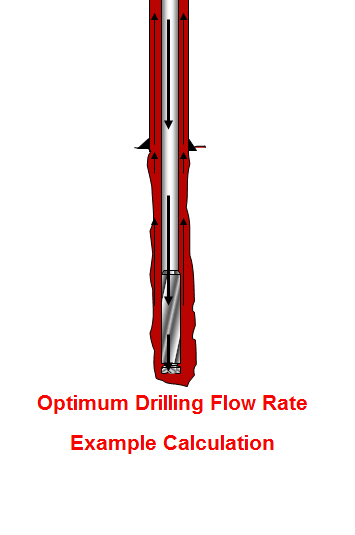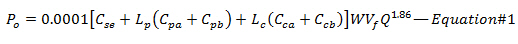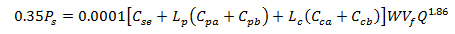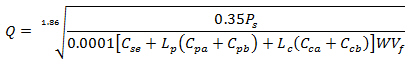## Optimum Drilling Flow Rate Example Calculation

This topic will demonstrate how to determine optimum drilling flow rate for both drilling hydraulic optimization methods (the maximum hydraulic horse power and the maximum impact force). If you don’t know the equations, please read this article first, Determine Optimum Drilling Flow Rate for Basic System – Drilling Hydraulics.The information is listed below:

Hole size = 8.5 inch

Depth In = 2,000 ft

Depth out = 6,000 ft

Mud properties at starting point

Mud weight = 9.2 ppg

PV = 13

YP = 10

## Determine Optimum Drilling Flow Rate for Basic System – Drilling Hydraulics

From the previous post (total pressure loss excluding the bit), we know the equation (shown below)We can solve the equation to get the flow rate (Q) that match with optimization criteria (Maximum Hydraulic Horsepower or Maximum Impact Force)

Maximum Hydraulic Horsepower will be happened when the pressure drop across the bit is equal to 65% of total pressure loss; therefore, 35% of total pressure loss (Ps) occurs through the drill stem (Po). The equation will be like this Po = 0.35 x Ps. By substituting Po with 0.35Ps into the Equation#1, you can solve for the optimum flow rate which will give you the maximum hydraulic horsepower.Solve for QMaximum Impact Force can be obtained when 48% of total pressure loss is at the bit so 52% of total pressure loss (Ps) occurs through the drill stem (Po). Substituting 52% of Ps into the Equation#1 will allow you to get the optimum flow rate at maximum impact force.Solve for Q